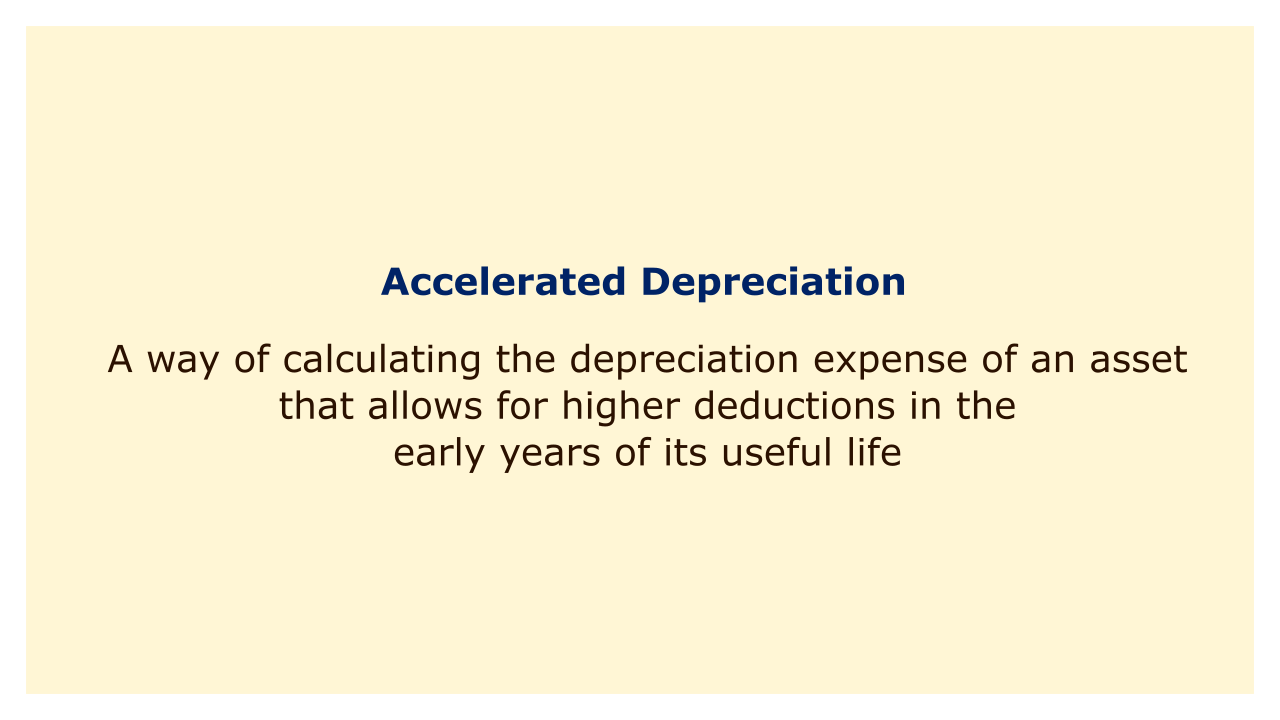# Accelerated DepreciationImage: Moneybestpal.com

### Accelerated depreciation is a method of calculating an asset's depreciation expense that permits greater deductions in the first few years of its useful life. As a result, the book value of the asset declines more quickly than it would under the straight-line method, which allots the same amount of depreciation each year.

The decision to adopt accelerated depreciation for a company's assets can be made for a variety of reasons. Given that depreciation is an expense that affects net income, one of them is to reduce the taxable income during the initial years. Another justification is to align depreciation costs with actual asset wear and tear, which is typically higher for more modern and effective assets.

The double-declining balance method and the sum of the years' digits approach are the two most widely used techniques for accelerated depreciation. Each approach calculates the depreciation expense by applying a percentage factor to the asset's book value at the start of the year.

A factor twice the straight-line rate is employed in the double-declining balance approach. The straight-line rate, for instance, is 10%, while the double-declining balance rate is 20% if an asset has a 10-year useful life. By multiplying 20% by the book value at the start of the year, the depreciation expense for each year is determined. Up until the asset's salvage value is reached, the book value is decreased by the depreciation expense annually.

The sum of the years' digits method uses a factor that is based on the remaining useful life of the asset divided by the sum of all years' digits. For instance, if an asset has a 10 year useful life, the total number of years is 55 (10 + 9 + 8 +... + 1). The book value at the start of the year is multiplied by a fraction with the remaining useful life as the numerator and 55 as the denominator to determine the depreciation charge for each year. For instance, the fraction is 10/55 in year 1, 9/55 in year 2, and so forth.

In both cases, depreciation costs rise in the initial years and fall in the subsequent years. An organization's financial statements and tax obligations may be significantly impacted by this. As a result, it's crucial to comprehend how accelerated depreciation functions and when it should be applied.
Tags